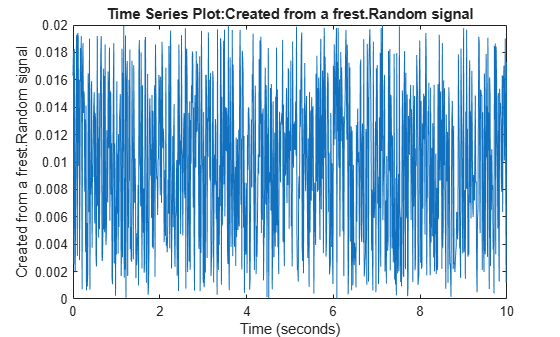frest.Random

Random input signal

Description

Use a frest.Random object to represent a random input signal for frequency response estimation. The random signal contains uniformly distributed random numbers in the interval [0 Amplitude] or [Amplitude 0] for positive and negative amplitudes, respectively.

Random signals are useful because they can excite the system uniformly at all frequencies up to the Nyquist frequency.

You can use a random input signal for estimation at the command line, in the Model Linearizer, or with the Frequency Response Estimator block. The estimation algorithm injects the signal at the input point you specify for estimation, and measures the response at the output point.

When you use a random input signal for estimation, the frequencies returned in the estimated frd model depend on the length and sampling time of the signal. They are the frequencies obtained in the fast Fourier transform of the input signal. For more information, see the Algorithm section of frestimate.

To view a plot of your input signal, type plot(input). To create a timeseries object for your input signal, use the generateTimeseries command.

Creation

Description

input = frest.Random(sys) creates a random signal with properties based on the dynamics of the linear system sys. For instance, if you have an exact linearization of your system, you can use it to initialize the parameters.

example

input = frest.Random(Name,Value) creates random signal with properties specified using one or more name-value pairs. Enclose each property name in quotes.

Input Arguments

expand all

Linear dynamic system, specified as a SISO ss, tf, or zpk object. You can specify known dynamics or obtain the linear model by linearizing a nonlinear system.

The resulting frest.Random object automatically sets the following properties based on the linear system:

• Ts is set such that the Nyquist frequency of the signal is five times the upper end of the frequency range to avoid aliasing issues.

• NumSamples is set such that the frequency response estimation includes the lower end of the frequency range.

The remaining properties use default values.

Properties

expand all

Signal amplitude, specified as a scalar. If Amplitude is:

• Positive, the random signal values are uniformly distributed in the range [0 Amplitude]

• Negative, the random signal values are uniformly distributed in the range [Amplitude 0]

Sample time of the random signal in seconds, specified as a positive scalar.

Number of samples in the random signal, specified as a positive integer.

This property does not determine number of frequency points in the final estimation result. The frestimate function discards any frequency points with negative values for this signal.

Random number stream, specified as a RandStream object. The state of the stream you specify is stored with the input signal. This stored state allows the software to return the same result every time you use generateTimeseries and frestimate with the input signal.

By default, Stream is the default stream of the current MATLAB® session.

Object Functions

 frestimate Frequency response estimation of Simulink models generateTimeseries Generate time-domain data for input signal frest.simCompare Plot time-domain simulation of nonlinear and linear models frest.simView Plot frequency response model in time- and frequency-domain getSimulationTime Final time of simulation for frequency response estimation

Examples

collapse all

Create a Random input signal with 1000 samples taken at 100 Hz and an amplitude of 0.02.

input = frest.Random('Amplitude',0.02,...
'Ts',1/100,...
'NumSamples',1000);

Plot the random signal.

plot(input)Create a multiplicative lagged Fibonacci generator random stream.

stream = RandStream('mlfg6331_64','Seed',0);

Create a random input signal using this stream.

input = frest.Random('Stream',stream);

Alternative Functionality

Model Linearizer

In the Model Linearizer, to use a random input signal for estimation, on the Estimation tab, select Input Signal > Random.

Introduced in R2009b